# What Is Compound Interest?9 min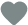171

Understanding how compounding interest works can help you to grow your wealth. Compound interest power requires patience and time. You will be able to see extraordinary results if you are ready to play a long game.

Start investing earlier - become richer faster. Nowadays, we can often hear that from everyone starting from amateur bloggers to experienced financial advisors. But what does it essentially mean?

Millions of people now have mobile apps that allow them to trade stocks as simple as sharing photos on social media. The number of retail investors worldwide boomed during the last years. The pandemic contributed to the spike of interest for millions of individuals. Many of them were attracted to the financial markets due to the so-called “magic of compounding”. The meaning of compound interest is simple - it is the interest you earn on your interest.

As we know, interest simply refers to the cost of capital paid by the borrowers to the lenders and it is expressed in percentage. It makes a whole lot of difference in the initial amount of money deposited, invested, or loaned out but the magnitude of the difference depends on the type of interest the money accrued.

## Compound Interest meaning

So, what is compound interest? It is the interest accrued from a principal amount and the initial interest on it. This means that compound interest sets to almost double an amount within a specified period given the stability of the market, time, and other factors because it is "interest on interest".

There are simple interest and compound interest. The difference between the two is that while the simple interest grows at a linear rate, the compound interest grows at an exponential rate which is not directly proportional to the initial amount. Compound interest seems more profitable to the investor.

The most prominent factor that determines compound interest is the time which is commonly referred to as the compounding period. It is the time rate in which the interest should increase.

The compounding period varies depending on the way the principal amount is put to use and the way it accrues its simple interest. If the principal amount is saved in the bank, the compound interest can be accrued daily. However, for P2P lending, the interest can be accrued any period, either daily, weekly, monthly, quarterly, or yearly.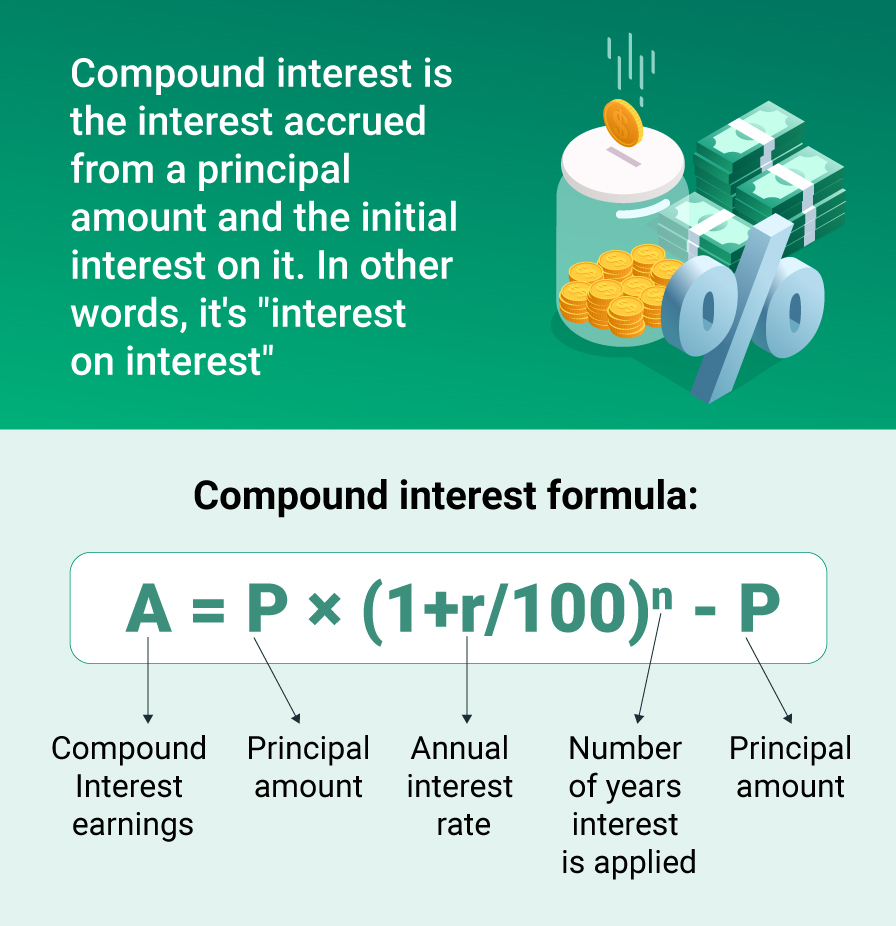## How does compound interest work?

If something compounds, it means that a little growth serves as the fuel for future growth. The extraordinary results of compounding are hard to underestimate although they seem counterintuitive.

It doesn’t seem so obvious to us since we are used to linear thinking rather than exponential thinking. It is easier to calculate 2+2+2+2+2 in your head than 2x2x2x2x2. Morgan Housel, in his famous book “Psychology of Money”, suggests the counterintuitiveness of compounding is responsible for the majority of disappointing trades, bad strategies, and successful investing attempts.

The compound interest rewards the patient ones. One of the most notable cases of compound interest is the case of Warren Buffet. Many of us don’t pay attention to one simple fact: he is not just a good investor, he was a good investor since he was literally a child and continues to be a phenomenal investor.

When discussing the compound interest and Warren Buffet, it is equally important to point out what he didn’t do. When investing consistently over the decades, he didn’t get carried away with debt, didn’t do panic-selling during the many recessions, didn’t damage the reputation of his business, didn’t become attached to the one strategy or trend, didn’t choose to retire too early. This consistency and longevity make compounding interest work.

## Compound Interest VS Simple Interest

There are two methods of calculating your interest which include compound and simple interest.
It is better to see it once than to hear about it a thousand times. In order to give a more representative example of how compounding interest works and its advantages, we will apply compound interest and simple interest formulas to the same amounts and the same interest rates and compare the results.

### How to calculate simple interest?

The simple interest rate is calculated by using the following interest rate formula:

S = P × (r/100) × n

where:

S = Simple interest
P = Principal amount
r = Annual interest rate
n = Term of loan, in years.

For instance, if you lend €100 for 5% annually, the interest you receive is simple, as it is the cost for the loan and the borrower will pay you the set fee in the amount of 5%. This interest is based on the principal amount - €100 - and your total will be €105 for the first year. After 10 years of getting simple interest from the €100, you are going to get €50 of profit, making it €150 in total.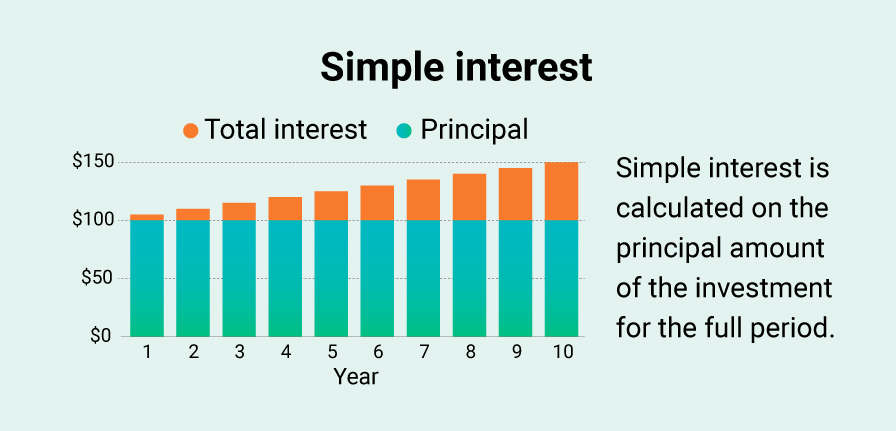Alternatively, the compound interest rate is based on the principal amount of €100 and the interest rate of 5% that accumulates on the principal amount in every period. Without monthly contributions, the initial €100 in ten years transforms into ~ €163 thanks to the power of compound interest.

We can see that compound interest considers the interest of the previous period. That is why you call it interest on interest. The calculation of compound interest is difficult compared to simple interest.

### How to calculate the compound interest?

Now let’s move to the part where the real magic of compounding starts and where the rich become even richer. The compounding interest can be calculated by using the compound interest formula:

A = P  × (1+r/100)n - P

where:

A = Compound Interest earnings
P = Principal amount
r = Annual interest rate
n = Number of years interest is applied

Take the same €100. By using basic math, investing €100, and earning 5% each year, you will have €105 at the end of the first year of investing. At the end of the second year of investing, your earned money will accrue an additional €0.25, and then your total will be €110.25.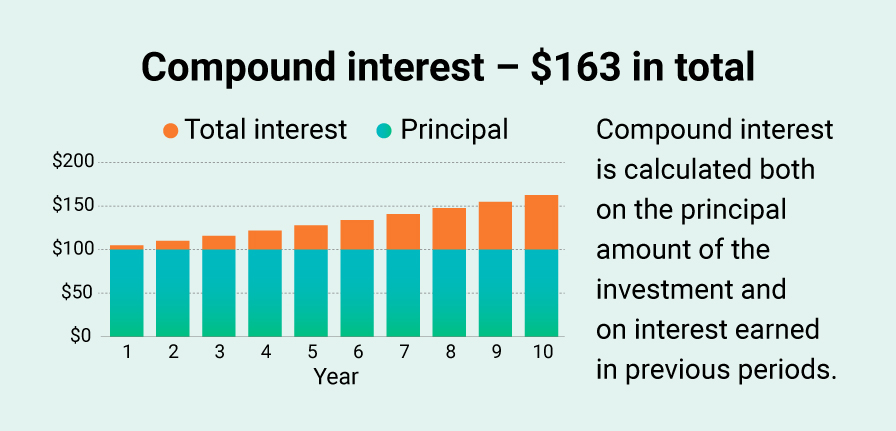## Examples of compound interest schedules

The compound interest can be calculated taking into account different frequencies. It can be accumulated on a daily, weekly, monthly, or annual basis. Standard compounding frequency plans are commonly used with traditional financial products. For instance, your savings account at the bank would typically have a daily compounding schedule. If you take mortgages, or personal business loans, credit card loans most probably will have a monthly compounding schedule.

There may be differences in the time it takes for the accrued interest to be credited to the existing balance. For example, the interest rate on your savings account at the bank may be compounded every day, but only credited once a month. Only after the interest is credited to your account, it begins to generate further interest and make your wealth grow. This is why uninterrupted compounding is important.

### Example 1

If compound interest is calculated annually, the formula would look like this:

C = P × (1+r/100)n - P

Mr. A invests €8,000 on a compound interest rate of 5% annually for a period of 6 years. Compound interest would be calculated thus:

In this case, P = €8,000, r = 5% and n = 6
Compound interest = 8,000 × (1+5/100)6 – 8,000 = 2,720.77

Therefore, the compound interest over 6 years is €2,720.77.

Meanwhile, if an investor decides to choose a simple interest option of the same 6 years period, we get this calculation:

Simple interest = 8,000 × (5/100) × 6 = 2,400

Therefore, an investor will receive only €2,400 simple interest instead of €2,720.77 compound interest.

### Example 2

If compound interest is on quarterly compounding, the formula would look like this:

Compound interest = P × (1+r/4×100)n×4 – P

Mr. A invests €8,000 on an annual interest rate of 5%, quarterly compounding for 6 years. Compound interest accrues quarterly and we get this calculation:

Compound interest =  8,000 × (1+5/4×100)6*4 – 8,000 = €2,778.81

### Example 3

If compound interest is monthly compounding, the formula would look like this:

Compound interest = P × (1+r/100×12)n×12 – P

Mr. A invests €8,000 on an annual interest rate of 5%, monthly compounding for a period of 6 years. Compound interest accrues monthly and we get this calculation:

Compound interest = 8,000 × (1+5/100×12)6*12 – 8,000 = €2,792.14

So we see that monthly compound interest has proven to be the most profitable.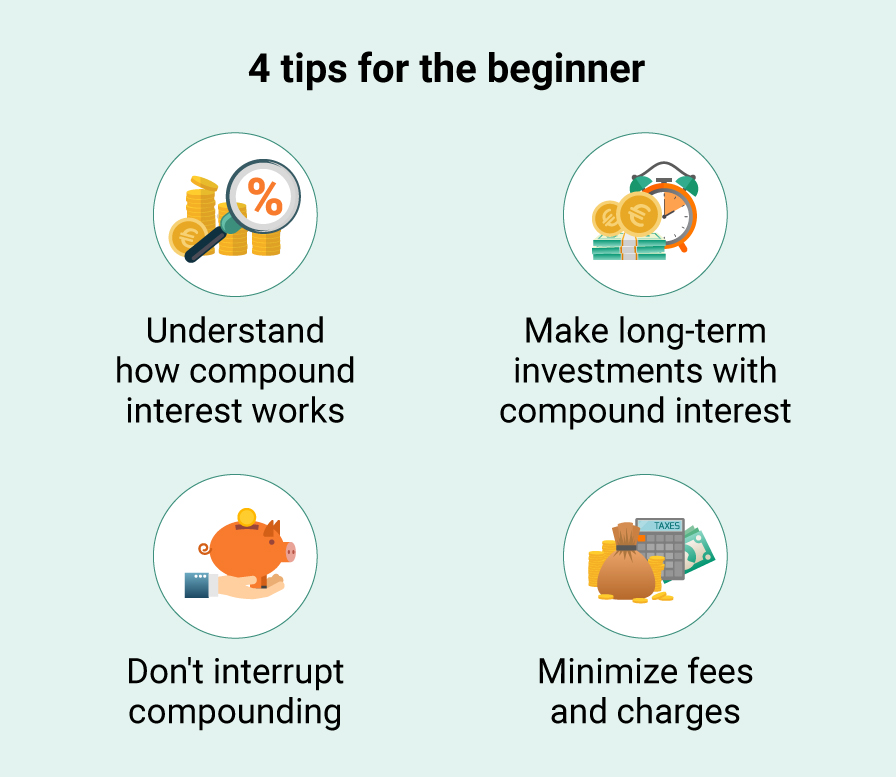## Why Is Compound Interest So Important?

When 5% per annum from the example above doesn’t seem so pleasant, we can’t blame people who are high-returns seekers. It is rather intuitive - to be attracted to the higher returns.

But what makes a good investment strategy? Compounding is the key.

Earning good and stable returns for a long period of time and reinvesting them, that’s where compounding shows its magic.

High-interest rates are attractive, however, a wise investor should always make a distinction between investing and speculation and mind the risks that always come together with higher returns. Good investment strategy takes patience and wisdom, the compounding magic cannot be interrupted. It can be compared to planting a tree. If you plant a tree today and come back to it in a year, you don’t expect meaningful progress, right? But when you come back five, ten, or even 30 years later you will be able to see extraordinary progress.

The compound interest requires that the investor does not withdraw the nominal interest but reinvests it together with the principal amount. The potential of compound interest to grow exponentially makes it a very good option for wealth creation.

Compounding will help your investment grow regardless of the initial amount you invested. You can start small and, by letting your money work for you, you can efficiently beat inflation and achieve higher returns by reinvesting your interest payments.

Another importance of compound interest is its ability to serve as a long-term investment. This is possible because of the ability of compound interest to double the initial amount within a given period. For instance, a parent who wants to establish a college fund for a child can invest an initial amount of €7,000 as soon as the child is born and leave it at 5% for 20 years. In 20 years, with compound interest, the initial amount would have doubled to €18,573 which is quite amazing.

As an investor, good and clear knowledge of compound interest is very essential as it gives you an idea of what you have to do, why you have to do it, and what exactly is at stake. Information on how to compute compound interest enables an investor to know the duration that investment can take before it reaches the expected target. Some investments are not best if compound interest is involved. For instance, compound interest might not be applicable if one seeks to invest to set up an emergency fund, but it can apply if an investor is looking to invest and save up a retirement fund.

## Compound interest in P2P Investing

Compound interest applies to a lot of areas like mutual funds, stocks, compound interest savings accounts, peer-to-peer investment, and other investment portfolios. However, a significant area where it applies is the peer to peer-peer investment which is also known as P2P investing. Peer-to-peer investment is a scheme that brings together potential investors who become lenders and links them up with borrowers who borrow money at a subsidized rate while providing guarantors or in some cases collateral before they can gain access to the money. The borrowers are often given a time frame to pay back with interest which serves as a gain to the investors.

Investing in P2P schemes offers both simple and compound interest rates. For the simple interest, a stipulated percentage is agreed to be paid to the investor who is the lender. This stipulated percentage can be paid out monthly or at the end of the fiscal year.

At times, P2P loans are amortized loans.  Amortization involves spreading the monthly payments such that at the end of the month, a part of the principal amount and interest is covered in the monthly payouts.

On Robo.cash the amortisation is actually favorable for investor. If you invest €1,000 for 12 months at 12%, you are set to get €120 profit. However, you get a part of the interest every month, while the principal remains invested. This interest is reinvested as well. After the year, you will have got the €1,000 + €120 “pure” interest + the interest generated from the monthly interest throughout the year.

The magic of compound interest has always been here and helped many investors on the stock market to become richer. In its turn, the P2P investing market is rather new to make 50 years projections for it, but the same compound interest formulas can be applied for it. If you consider higher interest rates and are ready to take the risks, the P2P investing market offers higher returns than the stock market on average. For instance, by investing on Robocash and reinvesting your earnings using our fully automated investment tool you can take advantage of compound interest and enable its power.

As compound interest magic works and your income grows over the years, the commissions and fees for the traditional banking or investment products can easily eat a big part of your total interest over the years and partially destroy the magic. Among the advantages of P2P investing on Robocash is commission-free investing. When the traditional product will probably need to re-think their pricing models soon, we do not charge and never charged any commissions on the income your investments generated on the platform.